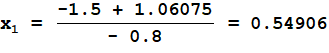### Full Width at Half Maximum (FWHM) & Quadratic equation

Full width at half maximum (FWHM) for a curve is used in many scientific experimental data analyses. For instance, in X–ray diffraction analysis of crystal data, it is used to find the grain size from the curve.

FWHM is the width of the curve or simply the difference in the corresponding two ‘x’ data values at ymax/2.

ymax refers the highest value of ‘y’ or ‘y’ value for the maximum peak height.

Example

Set of ‘x’ and ‘y’ data and the corresponding curve is given below:

x      y

0.0    0.000

0.1    0.146

0.2    0.284

0.3    0.414

0.4    0.536

0.5    0.650

0.6    0.756

0.7    0.854

0.8    0.944

0.9    1.026

1.0    1.100

1.1    1.166

1.2    1.224

1.3    1.274

1.4    1.316

1.5    1.350

1.6    1.376

1.7    1.394

1.8    1.404

1.9    1.406

2.0    1.400

2.1    1.386

2.2    1.364

2.3    1.334

2.4    1.296

2.5    1.250

2.6    1.196

2.7    1.134

2.8    1.064

2.9    0.986

3.0    0.900

3.1    0.806

3.2    0.704

3.3    0.594

3.4    0.476

3.5    0.350

3.6    0.216

3.7    0.074

3.8    -0.076The given data fits in the following equation: y = -0.4x2 + 1.5x.

In the above data the maximum value of y (ymax)is 1.406.

Hence, ymax/2 = 1.406/2 = 0.703.

Now, FWHM is the difference between the two ‘x’ values corresponding to this 0.703.

Let’s find out the two ‘x’ values corresponding to this ymax.

Since the curve fits in the equation,

y = -0.4x2 + 1.5x substitute y as 0.703.

0.703 = –0.4x2 + 1.5x

To find the value of ‘x’ for this ‘y’ value, quadratic equation can be used.

Rearrange the equation:

–0.4x2 + 1.5x – 0.703 = 0

It is in quadratic equation form:

ax2 + bx + c = 0

where ‘a’, ‘b’ and ‘c’ are constants.

The solution to this quadratic equation is given by the following quadratic formula:Here b2 – 4ac is called as discriminant.

If b2 – 4ac > 0, then the equation has two solutions.

If b2 – 4ac = 0, then the equation has one solution.

If b2 – 4ac < 0, then the equation has no real solutions.

Using quadratic equation, it is possible to find the value of ‘x’, if ‘a’, ‘b’ and ‘c’ are known.

In this, a = –0.4; b = 1.5; c = –0.703.

On substituting these values,So ‘x’ has two values.If these two ‘x’ values are substituted in the curve fitting equation,

y = -0.4x2 + 1.5x, then y = 0.703.

y = [-0.4 × 0.549062] + [1.5 × 0.54906] = 0.703.

y = [-0.4 × 3.200942] + [1.5 × 3.20094] = 0.703.

So, two ‘x’ values for the corresponding ymax/2 are: x2 = 3.2 and x1 = 0.55.

So, FWHM value for this curve is calculated as: (x2 – x1) = (3.2 – 0.55) = 2.65.

(Refer the curve above.)